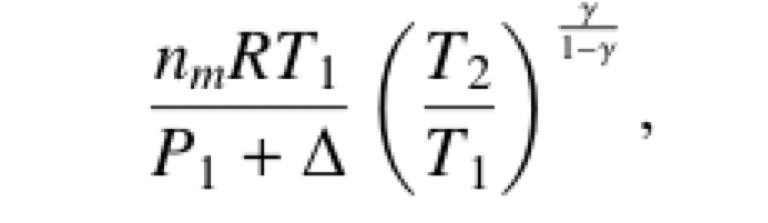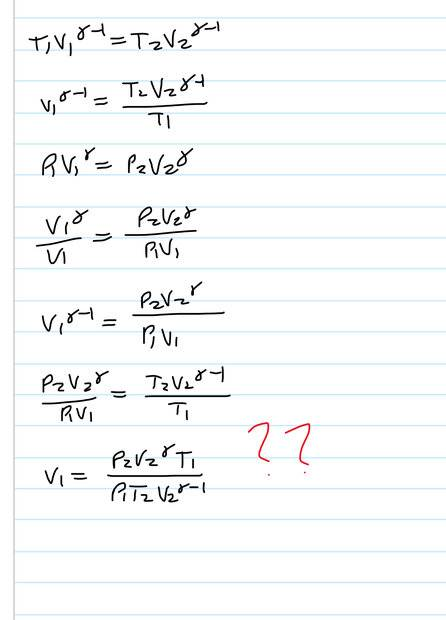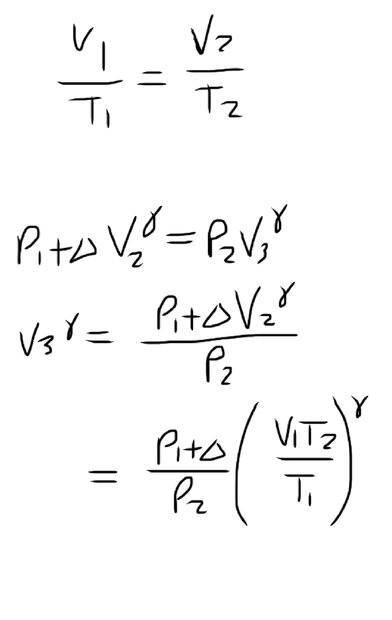# New volume of balloon after adiabatic expansion

so_gr_lo
Homework Statement:
A balloon has nm moles of monatomic ideal gas in thermal equilibrium with outside air at temperature T1. The pressure inside is P1+ delta. The ambient temperature increases isobarically from T1 to T2. The pressure then drops to P2 causing it to expand adiabatically until it’s temperature is again T1. Show that the new volume of the gas is (result shown in solution)

I seem to get T1 and T2 the wrong way round but can’t see how to fix it
Relevant Equations:
T1V1^(y-1) = T2V2^(y-1)

P1V1^y = P2V2^y
ResultMy attempt•Delta2

Homework Helper
Gold Member
2022 Award
I don't understand your first equation. What should happen to the volume if heat is added at constant pressure?

so_gr_lo
The first equation is for an adiabatic process. The volume should increase

Homework Helper
Gold Member
2022 Award
The first equation is for an adiabatic process. The volume should increase
Adiabatic? The ambient temperature increased to T2 at constant pressure, and as a result the temperature in the balloon increased, also, presumably, to T2. That's not adiabatic.
After that, the external pressure dropped abruptly causing the balloon to expand adiabatically.
Also, since ##\gamma=5/3>1##, your equation ##T_1V_1^{\gamma-1}=T_2V_2^{\gamma-1}## would have the volume decreasing as the temperature rises.

so_gr_lo
So I’ve changed the first equation, but now P1 + Δ and nRT1 are the wrong way round and I’ve got an extra V1 and don’t know where the ϒ/1-ϒ exponent comes from. V3 is the new volume.Homework Helper
Gold Member
2022 Award
So I’ve changed the first equation, but now P1 + Δ and nRT1 are the wrong way round and I’ve got an extra V1 and don’t know where the ϒ/1-ϒ exponent comes from. V3 is the new volume.

View attachment 301569
That all looks right, but you need to eliminate P2 so that you only have P1, V1, T1 and T2 on the right.

Gold Member
It may be just me but I am finding the notation rather confusing. I can see using 1 and 2 for changes of state but it would be nice if there were labels for outside and inside the balloon to be clear.

Homework Helper
Gold Member
2022 Award
It may be just me but I am finding the notation rather confusing. I can see using 1 and 2 for changes of state but it would be nice if there were labels for outside and inside the balloon to be clear.
Yes, there are a few confusing aspects to the notation. We have P2 corresponding to V3, not to V2, and ##P_1+\Delta## which might as well have been plain P1.

so_gr_lo
I can change the notation to Vfϒ = P1/Pf(V1Tf/T1)ϒ if that helps, where f is the final state. I still don’t think this is right because the answer has nmRT1 and this is equal to P1V1, but if I pull V1ϒ out the bracket I’ll have P1V1ϒ instead

Homework Helper
Gold Member
2022 Award
I can change the notation to Vfϒ = P1/Pf(V1Tf/T1)ϒ if that helps, where f is the final state. I still don’t think this is right because the answer has nmRT1 and this is equal to P1V1, but if I pull V1ϒ out the bracket I’ll have P1V1ϒ instead
As I wrote in post #6, you need to eliminate (what you are now calling) Pf.

so_gr_lo
If I use P1V2ϒ = PfVfϒ and rearrange for Pf, putting this in the equation I get Vfϒ = Vfϒ/V2ϒ(V1T2/T1)ϒ, but then the Vfϒ ‘s would cancel

Homework Helper
Gold Member
2022 Award
If I use P1V2ϒ = PfVfϒ and rearrange for Pf, putting this in the equation I get Vfϒ = Vfϒ/V2ϒ(V1T2/T1)ϒ, but then the Vfϒ ‘s would cancel
Yes, and that would get you back to ##V_1T_2=V_2T_1##, so that move is going backwards.
You have two equations, ##V_1T_2=V_2T_1## and ##P_1V_2^\gamma=P_fV_f^\gamma##. (The ##\Delta## is irrelevant; just call it P1.)
You need to eliminate ##P_f## and ##V_2##. Do you notice a difficulty? You will need another equation.
What given fact have you not used?

so_gr_lo
So I can rearrange to get V2 = V1T2/T1 and Pf= nmRT1V2ϒ/Vfϒ then substitute to get Pf = nmRT1(V1T2/T1)ϒ/Vfϒ

Homework Helper
Gold Member
2022 Award
So I can rearrange to get V2 = V1T2/T1 and Pf= nmRT1V2ϒ/Vfϒ then substitute to get Pf = nmRT1(V1T2/T1)ϒ/Vfϒ
Don’t worry about getting nmR in there yet. Concentrate on getting rid of ##P_f##.
What fact have you not used?? until it’s temperature is again T1

so_gr_lo
That it’s adiabatic between V2 and Vf, I thought PfVfϒ = P1V2ϒ was the equation for that?

Homework Helper
Gold Member
2022 Award
That it’s adiabatic between V2 and Vf?
No, that the final temperature is the original temperature: "until its temperature is again T1"

so_gr_lo
So could I do Pf = P1V2T1/ T2Vf

Homework Helper
Gold Member
2022 Award
So could I do Pf = P1V2T1/ T2Vf
Yes.

so_gr_lo
So substituting that I’d have Vfϒ-1 = P1T1(V1T2/V1)ϒ/V1T2

Homework Helper
Gold Member
2022 Award
So substituting that I’d have Vfϒ-1 = P1T1(V1T2/V1)ϒ/V1T2
At last you have the right collection of variables, but it does not look right. It's late here. Please post your working and I'll look at it in the morning.

so_gr_lo
I think what I posted above was incorrect here is my working

Pf = P1V2T1/T2Vf

Vf = P1(V1T2/T1)ϒ/Pf

= P1T2Vf(V1T2/T1)ϒ/V2T1

Vfϒ-1 = P1T2(V1T2/T1)ϒ/V2T1

using V2T1=V1T2

Vfϒ-1 = P1(V1T2/T1)ϒ/V1

as the T2 cancels

Homework Helper
Gold Member
2022 Award
Vfϒ-1 = P1(V1T2/T1)ϒ/V1

as the T2 cancels
I get the same answer, but we both get ##\gamma-1## where the solution quoted has ##1-\gamma##. It must be a typo.
Fwiw, here's how I solved it:
1. Isobaric stage: ##V_1T_2=V_2T_1##